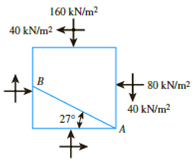Chapter 10, Problem 10.5PPrinciples of Geotechnical Enginee...

9th Edition
Braja M. Das + 1 other
ISBN: 9781305970939

Solutions

Chapter
SectionPrinciples of Geotechnical Enginee...

9th Edition
Braja M. Das + 1 other
ISBN: 9781305970939
Textbook Problem

A soil element is shown in Figure 10.38. Using the pole method, determine:a. Maximum and minimum principal stressesb. Normal and shear stresses on plane ABFigure 10.38

(a)

To determine

Calculate the maximum and minimum principal stresses using the pole method.

Explanation

Given information:

The normal stress along x axis (σx) is 80kN/m2.

The normal stress along y axis (σy) is 160kN/m2.

The shear stress along xy axis (τxy) is 40kN/m2.

The plane AB inclined at an angle of 27°.

Calculation:

Apply the procedure to construct the Mohr’s circle as shown below.

• Find the centre of the circle O1 located σx+σy2 from the origin.
• Find the radius (R) of the Mohr’s circle [σyσx2]2+τxy2.
• Sketch the Mohr’s circle once R has been determined.
• Draw a line CP parallel to the plane. Point P is the pole.
• Draw a line PD parallel to the plane AB. The point of intersection with Mohr’s circle is D.
• The coordinates of Q give the stresses on the plane AB.

Sketch the Mohr’s circle for the soil element as shown in Figure 1.

Refer to Figure 1.

Calculate the centre of the Mohr’s circle from the origin (OO1) as shown below.

OO1=σx+σy2

Substitute 80kN/m2 for σx and 160kN/m2 for σy.

OO1=80+1602=2402=120kN/m2

Calculate the radius (R) Mohr’s circle as shown below.

R=[σyσx2]2+τxy2

Substitute 80kN/m2 for σx, 160kN/m2 for σy, and 40kN/m2 for τxy

(b)

To determine

Calculate the normal and shear stresses on plane AB using the pole method.

Still sussing out bartleby?

Check out a sample textbook solution.

See a sample solution

The Solution to Your Study Problems

Bartleby provides explanations to thousands of textbook problems written by our experts, many with advanced degrees!

Get Started

What are the major components of a bicycle?

Engineering Fundamentals: An Introduction to Engineering (MindTap Course List)

Describe data and process modeling concepts and tools.

Systems Analysis and Design (Shelly Cashman Series) (MindTap Course List)

What will Iris have on her to-do list?

Management Of Information Security

Use A992 steal and. select a W shape. a. Use LRFD. b. Use ASD.

Steel Design (Activate Learning with these NEW titles from Engineering!)

Describe the uses of tags. List steps to tag digital content.

Enhanced Discovering Computers 2017 (Shelly Cashman Series) (MindTap Course List)